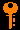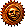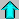# Lecture 5 - Orbital Mechanics I (9/24/98)Newton --- | --- BarycenterChapter 1-4, 1-5, P1-7 (ZG4)

Isaac Newton
Key Question:## Investigations:

1. Steps Toward Universal Graviatation
• What does Kepler's 3rd Law imply for the circular velocity?
• What does the centripetal acceleration plus Kepler's 3rd imply for the gravitational acceleration in a circular orbit?
• What is the form of the gravitational force in a circular orbit?
• What does Newton's 3rd Law imply about the form of the gravitational force law and its dependence on the masses of the two bodies?
• Why is Newton's gravitational force law "universal"?
• If the forces on the two bodies under mutual gravitation are equal due to Newton's 3rd Law, what does this imply about the respective accelerations?
2. Acceleration and Weight
• What is the gravitational force at the surface of or outside a spherical mass distribution equal to?
• What do we mean by the surface gravitational acceleration g?
• What is the difference between the gravitational acceleration at the distance of the moon scaled from g on the Earth, and the centripetal acceleration computed from the Moon's orbital period and orbital distance?
• What do we mean by the weight of an object?
• What is the difference between "weighing" 1 kg, and a mass of 1 kg?
• What is your weight on the surface of the moon?
• What do we mean when we say that you are "weightless" in space, such as in the low Earth orbit of the Space Shuttle (500km)?
3. Kepler's Laws Revisited I
• What do we mean by Keplerian rotation?
• How is Keplerian rotation different than solid body rotation (like a phonograph record)?
• How is a "skinny triangle" useful in the derivation of Kepler's 2nd law of equal areas?
• What are polar coordinates and why are they useful in orbits?
• What is angular momentum?
• Why is angular momentum conserved in an orbit?
4. Orbits Explained
• How does the motion of an orbiting satellite differ from the motion of a freely falling apple?
• What was Newton's modification to Kepler's Third Law?
• How can one determine the masses of mutually orbiting bodies?

## Infinitesimal Steps and Numerical Simulations

In the previous lecture, we used the notion of "infinitesimals" in the calculus of derivatives to derive the purely geometric centripetal acceleration when applied to a circular orbit. This is of more than academic interest, in that in systems more complicated than two mutually gravitating bodies the only method for solution is by numerically simulating the motions of the bodies with finite steps - essentially what we have done in our simple derivation!

To recap, we found that if we start at time t=t_0 at the position and velocity given by:

t = t0
x = r
y = 0
vx = 0
vy = v

After a time-step dt (technically delta-t) we have

t = t0 dt
x = r dx = - a dt2 / 2
y = 0 dy = v dt
vx = 0 dvx = a dt
vy = v dvy = 0

And thus after our single time-step dt we have the updated position

t = t0 dt t = t0 + dt
x = r dx = - a dt2 / 2 x = r - a dt2 / 2
y = 0 dy = v dt y = v dt
vx = 0 dvx = a dt vx = a dt
vy = v dvy = 0 vy = v

Note that this is only valid in the limit that dt -> 0, in that we have assumed that dx uses the average value of v_x over the step, and that dv_y = 0. These are both equivalent to the linear approximation in that we assume that the velocities grow linear with time under the approximation of a constant force over the timestep in the -x direction. There are higher order corrections to this which would include the changing aspect of the force in the movement.

What errors does this introduce? We noted that to remain on the circle x^2 + y^2 = r^2, the position after the time step must be given by

( r - a dt2 / 2 )2 + ( v dt )2 = r2

or after expanding the squares on the left hand side

r2 - r a dt2 + a2 dt4 / 4 + v2 dt2 = r2.

Cancelling the r^2 on each side, dividing through by -r dt^2, and isolating a on the left side, we get the useful expression

a = v2 / r + a2 dt2 / 4r.

If we take the ananlytic limit dt->0 then we get the usual expression for the centripetal acceleration

a = v2 / r

required to keep the body in a circular orbit. We can therefore think of the extra term

da = a2 dt2 / 4r

as an error term which scales as the square of the timestep. If we were to use this simple scheme to update (x,y) as above, we would make errors that would slowly compond, as the act like a force error. (Q: How does this error in acceleration translate to errors in the velocity (v_x,v_y) and eventually position (x,y)?)

This simple finite step scheme, with some upgrading, is essentially what is used in astrophysics when we want to numerically model systems with more than 2 bodies! This is called the N-Body problem, where the N refers to the arbitrariness of the number of bodies or particles in the system. Currently, we routinely run simulations with N=10^6 particles, and are pushing hard on simulations with N=10^8! Whew.

For an example of N-body simulations in cosmology, see the NCSA "Cosmos in a Computer" Expo.Prev Lecture ---Next Lecture ---Astr11 Index ---Astr11 Home

smyers@nrao.edu Steven T. Myers